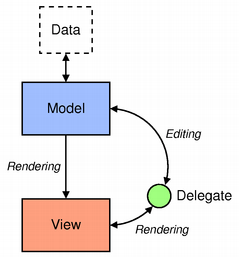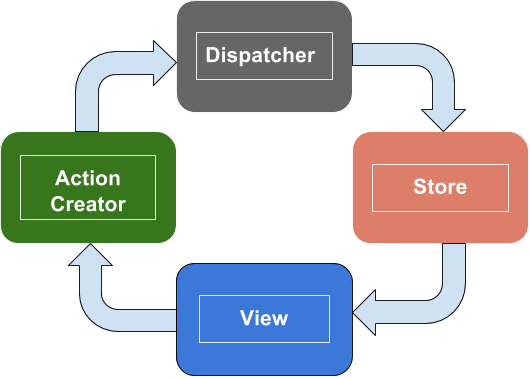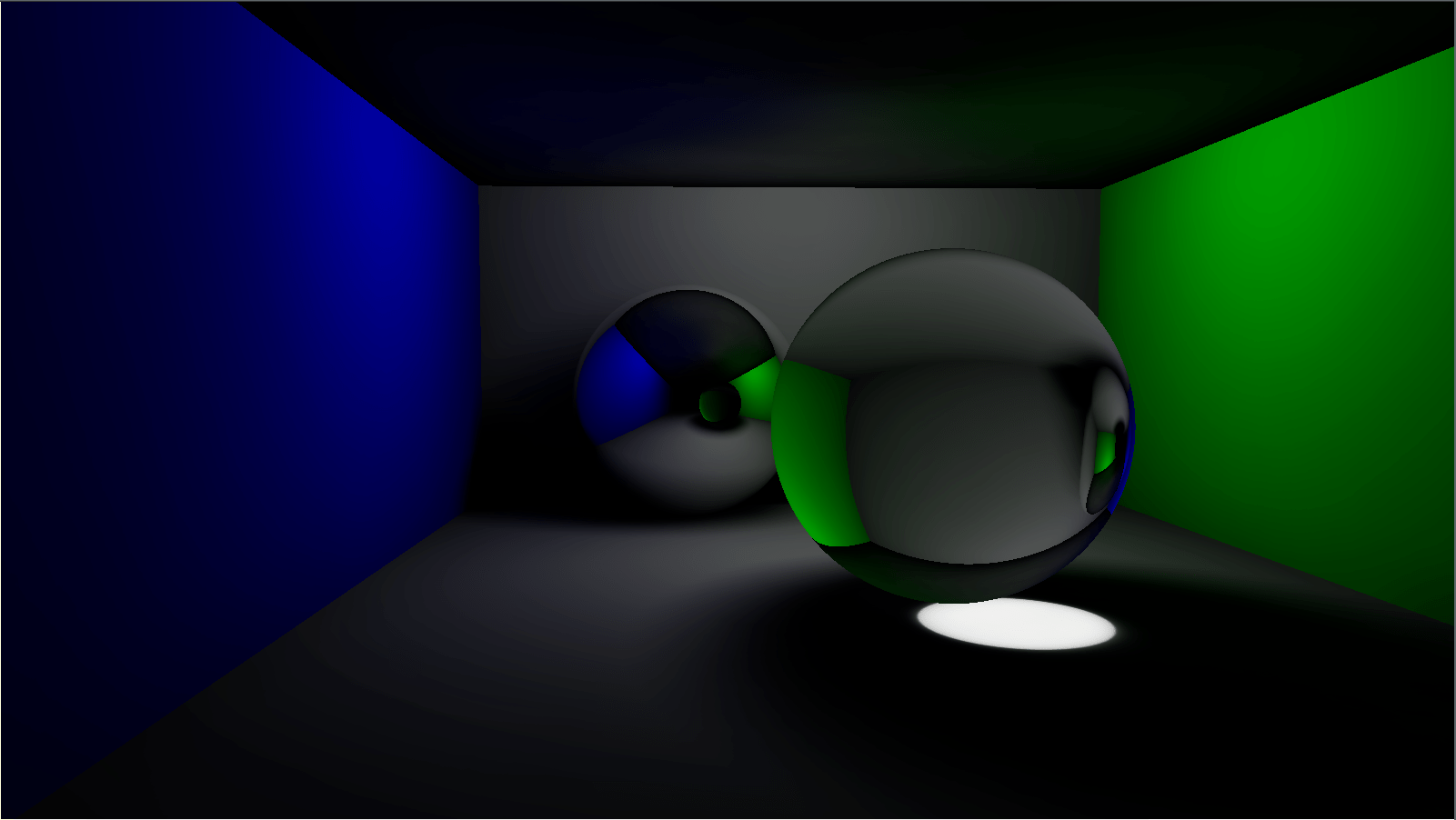# Flux: Qt Quick with unidirectional data flow

Hello !

Today is an important day, is the day of the first article of Qt I wrote.

Have you ever heard anything about Model View Controller or Model View Delegate???? Yes obviously, but right now, we’re going to talk about another thing (yes I am funny I know) . We are going to talk about Facebook, I mean a pattern which comes from Facebook.

# What are we going to talk about??

We are going to talk about the Flux pattern, this pattern says data flow should be unidirectional as opposed to the Model View Delegate pattern.Model View Delegate multi directional data flow : Credit QtFlux unidirectional data flow

### What is the advantages to use Flux pattern?

1. Signals propagation is easy and do not require any copy and paste.
2. The code is easy to read.
3. There is low coupling

### What is the Action Creator?

When a user wants to interact with the application, he want to do an “Action“. For example, he could wants to add things to a todo list, so he could launch an Action(“Things to do”);
The Action Creator is here to give Action to our dispatcher.

### What is the Dispatcher?

A dispatcher takes an action and its arguments and dispatchs it through all stores.

### What is the Store?

A store is like a collection of datas but it also has logic buried inside it.

### What is the View?

It shows all data.

### What are we going to see?

We are going to see how to write a little application using Flux.
Our application could seem to that :#### Let’s code !

First, we need to know what our application has to do.
It must have possibility to add a counter, increment and decrement them. We exactly have 3 actions. It is that simple !

Now, we are going to see what is the AppDispatcher.

A dispatcher should take as argument the action type and a message (id for example). This dispatcher should dispatch this action as well.

You easily could improve the dispatch behaviour. Indeed, it could be more safe to use a queue… But in this example, I just show the mechanism and try to don’t overcomplicate the app.

I remind dispatcher dispatchs through the stores.
A store is a singleton which manages all objects of the same type. Our store manages all counters in the app.

A counter is an object with an id and a value, knowing that, we easily get :

On the onDispatched check if it is the good action, and if it is, do the required work.

Now we just need a view, as you see before, we use a “model” to store  all data, it will be the same for the view / delegate, but even if we use model view delegate, we will keep a unidirectional data flow.

The delegate will explains how an item should be rendered.
It is componed by 2 buttons (+ and -) and a value :

Yeah I know, there is some duplication of code, it is not good…
Now, we have the possibility to render items, we should print many of them.
Flux tells us datas are coming from Store, so let’s implement what Flux says!

It is the end, if you have any questions, please, let me know !
Hope you enjoyed it and learned somethings !

Thanks !

# How to make a Photon Mapper : Photons everywhereBleed Color and Caustics.

Hello,

It has been a long time since I posted anything on this blog, I am so sorry about it.
I will try to diversify my blog, I’ll talk about C++, Rendering (always <3), and Qt, a framework I love.

So, in this last part, we will talk about photons.

## What exactly are Photons ?

A photon is a quantum of light. It carries the light straightforwardly on the medium. Thanks to it, we can see objects etc.

## How could we transform photons in a “visible value” like RGB color ?

We saw that the eyes only “see” the radiance !
So we have to transform our photons in radiance.

### From physic of photons

We know that one photon have for energy :$\displaystyle{}E_{\lambda}={h\nu}=\frac{hc}{\lambda}$

where$\lambda$ is the wavelength in nm and$E$ in Joules.
Say we have$n_\lambda$ photons of$E_{\lambda}$ each.
We can lay the luminous energy right now :$\displaystyle{Q_{\lambda}=n_{\lambda}E{\lambda}}$

The luminous flux is just the temporal derivative about the luminous energy :$\displaystyle{\phi_{\lambda}=\frac{dQ_{\lambda}}{dt}}$

The idea is great, but we have a luminous flux function of the wavelength, but the radiance is waiting for a general luminous flux
So, we want to have a general flux which is the integral over all wavelengths in the visible spectrum of the$\lambda$ luminous flux.$\displaystyle{\phi=\int_{380}^{750}d\phi_{\lambda}=\int_{380}^{750}\frac{\partial \phi_{\lambda}}{\partial\lambda}d\lambda}$

Now, we have the radiance$\displaystyle{L=\frac{d^2 \phi}{cos \theta dAd\omega}=\frac{d^2(\int_{380}^{750}\frac{\partial \phi_{\lambda}}{\partial \lambda}d\lambda)}{cos(\theta)dAd\omega}=\int_{380}^{750}\frac{d^3\phi_{\lambda}}{cos(\theta)dAd\omega d\lambda}d\lambda}$

Using the rendering equation, we get two forms :$\displaystyle{L^O=\int_{380}^{750}\int_{\Omega^+}fr(\mathbf{x}, \omega_i,\omega_o,\lambda)\frac{d^3\phi_{\lambda}}{dAd\lambda}d\lambda}$$\displaystyle{\int_{\Omega^+}fr(\mathbf{x}, \omega_i,\omega_o)\frac{d^2\phi}{dA}}$

The first one take care about dispersion since the second doesn’t.
In this post, I am not going to use the first one, but I could write an article about it latter.

## Let’s make our Photon Mapper

### What do we need ?

We need a Light which emits photons, so we could add a function “emitPhotons” .

We also need a material which bounces photons :

Obviously, we also need a structure for our photons. This structure should be able to store photons and compute irradiance at a given position.

### How could we do this ?

Photon emitting is really easy :

We divide the total flux by the number of photons and we compute a random direction, then we could trace the photon

Bouncing a photon depends on your material :

To take care about conservation of energy, we play Russian roulette.
Obviously, to take care about conservation of energy, we have to modify the direct lighting as well ^^.

Finally, we need to compute the irradiance at a given position :
It is only :$\displaystyle{E=\sum \frac {\phi}{\pi r^2}}$

So we could easily write :

To have shadows, you could emit shadow photons like this :

That’s all ! If you have any question, please, let me know !